TensorFlow Agents · TensorFlow Lite ·Building a board game app with TensorFlow: a new TensorFlow Lite reference app
outubro 29, 2021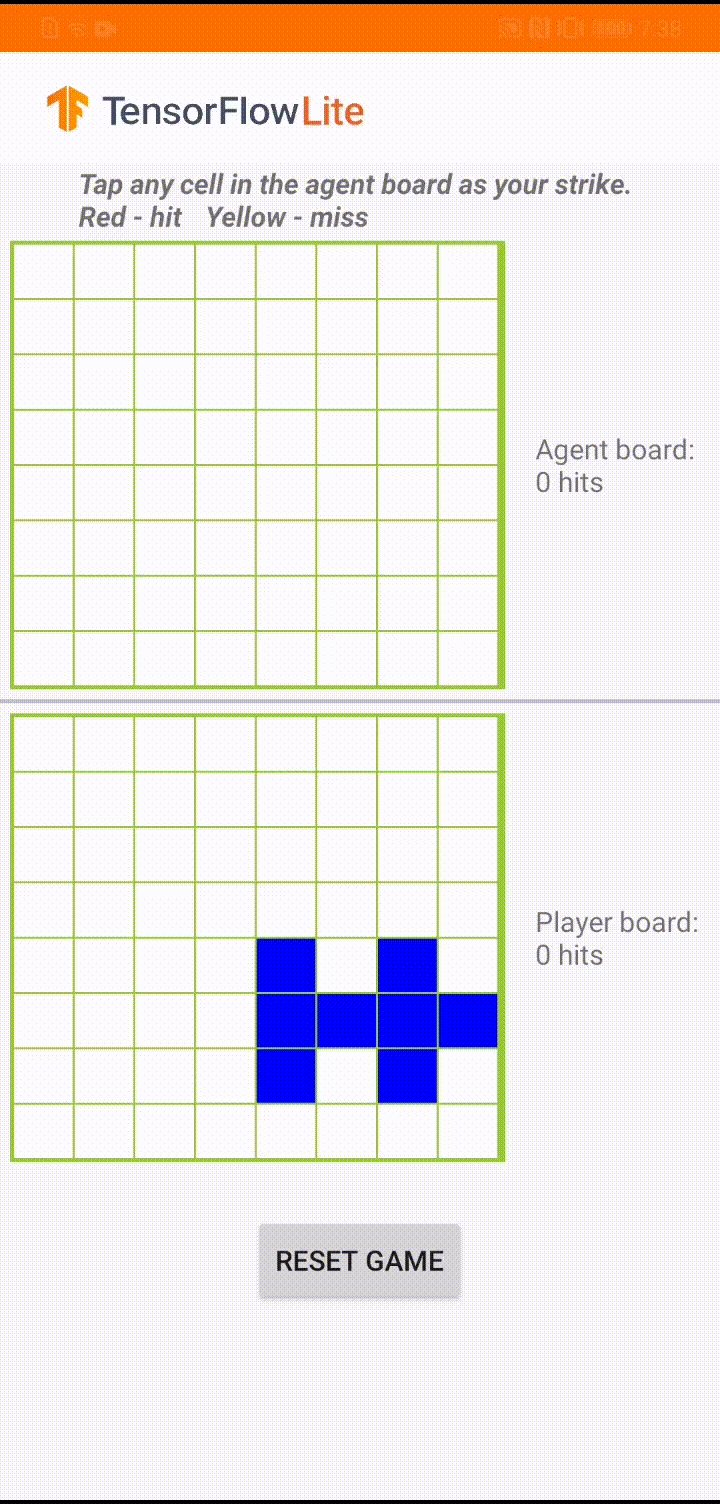Posted by Wei Wei, Developer AdvocateGames are often used as test grounds for various reinforcement learning (RL) algorithms. While it is very exciting that machine learning researchers invent new RL algorithms to master challenging games, we are also curious to see that game developers are using RL to build gaming bots in TensorFlow for various purposes, such as quality testing, game balance tuning and game difficulty assessment.

We already have a detailed tutorial that demonstrates how to implement the actor-critic RL method for the classical CartPole gym environment with TensorFlow. In this end-to-end tutorial, we are going to show you how to use TensorFlow core, TensorFlow Agents and TensorFlow Lite to build a game agent to play against a human user in a small board game app. The end result is an Android reference app that looks like below, and we have open sourced all the code in tensorflow/examples repository for your reference.Demo game play in ‘Plane Strike’

The game is called ‘Plane Strike’, a small board game that resembles the board game ‘Battleship’. The rules are very simple:

• At the beginning of the game, the user and the agent each have a ‘plane’ object (8 blue cells that form a ‘plane’ as you can see in the animation above) on their own boards; these planes are only visible to the owners of the board and hidden to their opponents.
• The user and the agent take turns to strike at one cell of each other’s board. The user can tap any cell in the agent’s board, while the agent will automatically make the choice based on the prediction of a machine learning model. The attempted cell turns red if it is a ‘plane’ cell (‘hit’); otherwise it turns yellow (‘miss’).
• Whoever achieves 8 red cells first wins the game; then the game is restarted with fresh boards.

Even though it may be possible to create handcrafted rules for such a small game, we turn to reinforcement learning to create a smart agent that a human player can’t easily beat. For a general introduction of reinforcement learning, please refer to this RL course from DeepMind and UCL.

We provide 2 paths of training and deployment for this game app

### TensorFlow Lite with a Python model written from scratch

In this path, to train the agent, we first create a custom OpenAI gym environment ‘PlaneStrike-v0’, which allows us to easily roll out game plays and gather game logs. Then we use the reward-to-go policy gradient algorithm to train the agent. REINFORCE is a policy gradient algorithm in RL. Its basic idea is to adjust the policy network parameters based on the reward signals collected during the gameplay, so that the policy network can maximize the return in future plays.

Mathematically, the policy gradient is defined as:

where:

• T: the number of timesteps per episode, which can vary per episode
• st: the state at timestep t
• at: chosen action at timestep t given state s
• πθ: is the policy parameterized by θ
• R(*): is the reward gathered, given the policy

Please refer to this DeepMind lecture on policy gradient for a more detailed discussion. To implement it with TensorFlow, we define a simple 3-layer MLP as our policy network, which predicts the agent’s next strike position, given the human player’s board state. Note that the log expression of the above policy gradient without the reward part is the equivalent of negative cross entropy loss. In this case, since we want to maximize the rewards, we can just minimize the categorical cross entropy loss to achieve that.

``model.compile(loss='sparse_categorical_crossentropy', optimizer=sgd)``

We create a play_game() function to roll out the game and help us gather game logs. After each episode, we train the agent via Keras fit() function:

``model.fit(x=board_log, y=action_log, sample_weight=rewards)``

Note that we pass the discounted rewards-to-go as ‘sample_weight’ into the Keras fit() function as a shortcut, to implement the policy gradient algorithm without writing a custom training loop. An intuitive way to think about this is we need a tuple of (x, y, reward) instead of just (x, y) as in supervised learning. Rewards, which can be negative, help the predictor output move toward/away from y, based on x. This is different from supervised learning (in which case your 'sample_weight' can never be negative).

Since what we are doing isn’t supervised learning, we can’t really use training loss to monitor the training progress. Instead, we are going to use a proxy metric ‘game_length’, which indicates how many steps the agent takes to finish each episode. Intuitively you can understand that if the agent is smarter and makes better predictions, the game length becomes shorter.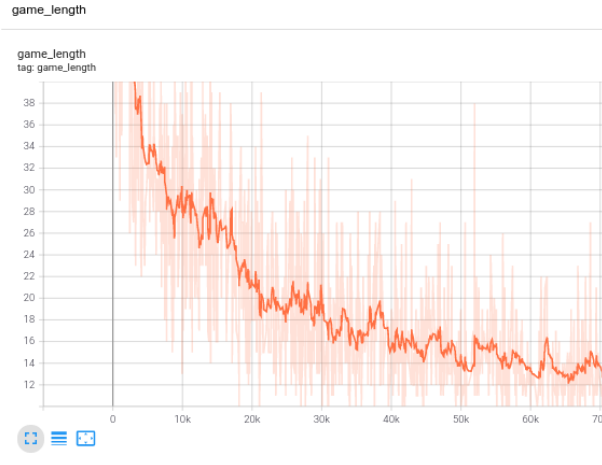Training progress in TensorBoard

Since this is a game that needs instantaneous responses from the agent, we want to deploy the model on mobile devices instead of servers. After training the model, we use the TFLite converter to convert the Keras model into a TFLite model, and integrate it into our Android app.

``````converter = tf.lite.TFLiteConverter.from_keras_model(model)
tflite_model = converter.convert()``````

The exported model is very fast and takes <1 ms to execute on Pixel phones. During the game play, at each step the agent looks at the user’s board position and predicts its next strike position to achieve 8 red cells as fast as possible.

``````convertBoardStateToByteBuffer(board);
tflite.run(boardData, outputProbArrays);
float[] probArray = outputProbArrays;
int agentStrikePosition = -1;
float maxProb = 0;
for (int i = 0; i < probArray.length; i++) {
int x = i / Constants.BOARD_SIZE;
int y = i % Constants.BOARD_SIZE;
if (board[x][y] == BoardCellStatus.UNTRIED && probArray[i] > maxProb) {
agentStrikePosition = i;
maxProb = probArray[i];
}
}``````

### TensorFlow Lite with a model trained with TensorFlow Agents

While it’s a good exercise to write our agent from scratch using TensorFlow API, it’s better to leverage existing implementations of RL algorithms. TensorFlow Agents is a library for reinforcement learning in TensorFlow, and makes it easier to design, implement and test new RL algorithms by providing well tested modular components that can be modified and extended. TF Agents has implemented several state-of-the-art RL algorithms, including DQN, DDPG, REINFORCE, PPO, SAC and TD3. Trained policies by TF Agents can be converted to TFLite directly and deployed into mobile apps (note that this feature is only recently enabled so you will need the nightly builds of TensorFlow and TensorFlow Agents).

We use the TF Agents REINFORCE agent to train our agent. First, we need to define a TF Agents training environment as we did with the gym environment in the previous section. Then we can define an actor net as our policy network

``````

actor_net = tfa.networks.Sequential([
tfa.keras_layers.InnerReshape([BOARD_SIZE, BOARD_SIZE], [BOARD_SIZE**2]),
tf.keras.layers.Dense(FC_LAYER_PARAMS, activation='relu'),
tf.keras.layers.Dense(BOARD_SIZE**2),
tf.keras.layers.Lambda(lambda t: tfp.distributions.Categorical(logits=t)),
],        input_spec=train_py_env.observation_spec(
))``````

We are going to use the built-in REINFORCE agent that TF Agents has already implemented. The agent is built on top of the ‘actor_net’ defined above:

``````  tf_agent = reinforce_agent.ReinforceAgent(
train_env.time_step_spec(),
train_env.action_spec(),
actor_network=actor_net,
optimizer=optimizer,
normalize_returns=True,
train_step_counter=train_step_counter)``````

To train the agent, we need to collect some trajectories as experience. We define a function just for that using DeepMind Reverb and TF Agent PyDriver:

``````def collect_episode(environment, policy, num_episodes, replay_buffer_observer):
"""Collect game episode trajectories."""
initial_time_step = environment.reset()

driver = py_driver.PyDriver(
environment,
py_tf_eager_policy.PyTFEagerPolicy(policy, use_tf_function=True),
[replay_buffer_observer],
max_episodes=num_episodes)
initial_time_step = environment.reset()
driver.run(initial_time_step)``````

Now we are ready to train the model:

``````for i in range(iterations):
# Collect a few episodes using collect_policy and save to the replay buffer.
collect_episode(train_py_env, collect_policy,
COLLECT_EPISODES_PER_ITERATION, replay_buffer_observer)

# Use data from the buffer and update the agent's network.
iterator = iter(replay_buffer.as_dataset(sample_batch_size=1))
trajectories, _ = next(iterator)
tf_agent.train(experience=trajectories)
replay_buffer.clear()``````

You can monitor the training progress using TensorBoard. In this case, we visualize both the average episode length and average return.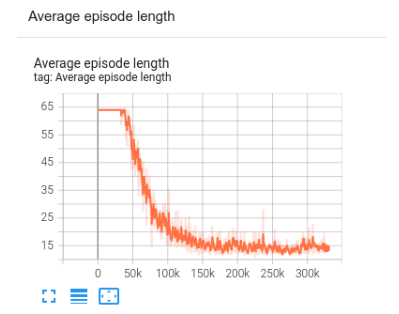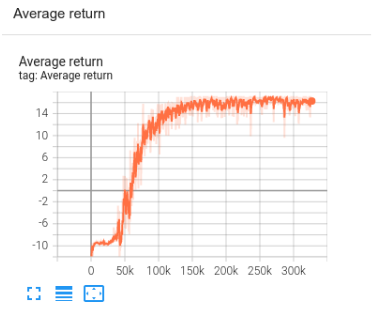TF Agents training progress in TensorBoard

Once the policy has been trained and exported as SavedModel, you can converted it into a TFLite model:

``````converter = tf.lite.TFLiteConverter.from_saved_model(
policy_dir, signature_keys=['action'])
converter.target_spec.supported_ops = [
tf.lite.OpsSet.TFLITE_BUILTINS,  # enable TensorFlow Lite ops.
tf.lite.OpsSet.SELECT_TF_OPS  # enable TensorFlow ops.
]
tflite_policy = converter.convert()
with open(os.path.join(model_dir, 'planestrike_tf_agents.tflite'), 'wb') as f:
f.write(tflite_policy)``````

Currently there are a few TensorFlow ops that are required during the conversion. The converted model is slightly different from the model we trained using TensorFlow directly, because it takes 4 tensors as the input. What really matters here is the ‘observation’ tensor. Our agent will look at this ‘observation’ tensor and predict its next move. The other 3 can be safely ignored at inference time.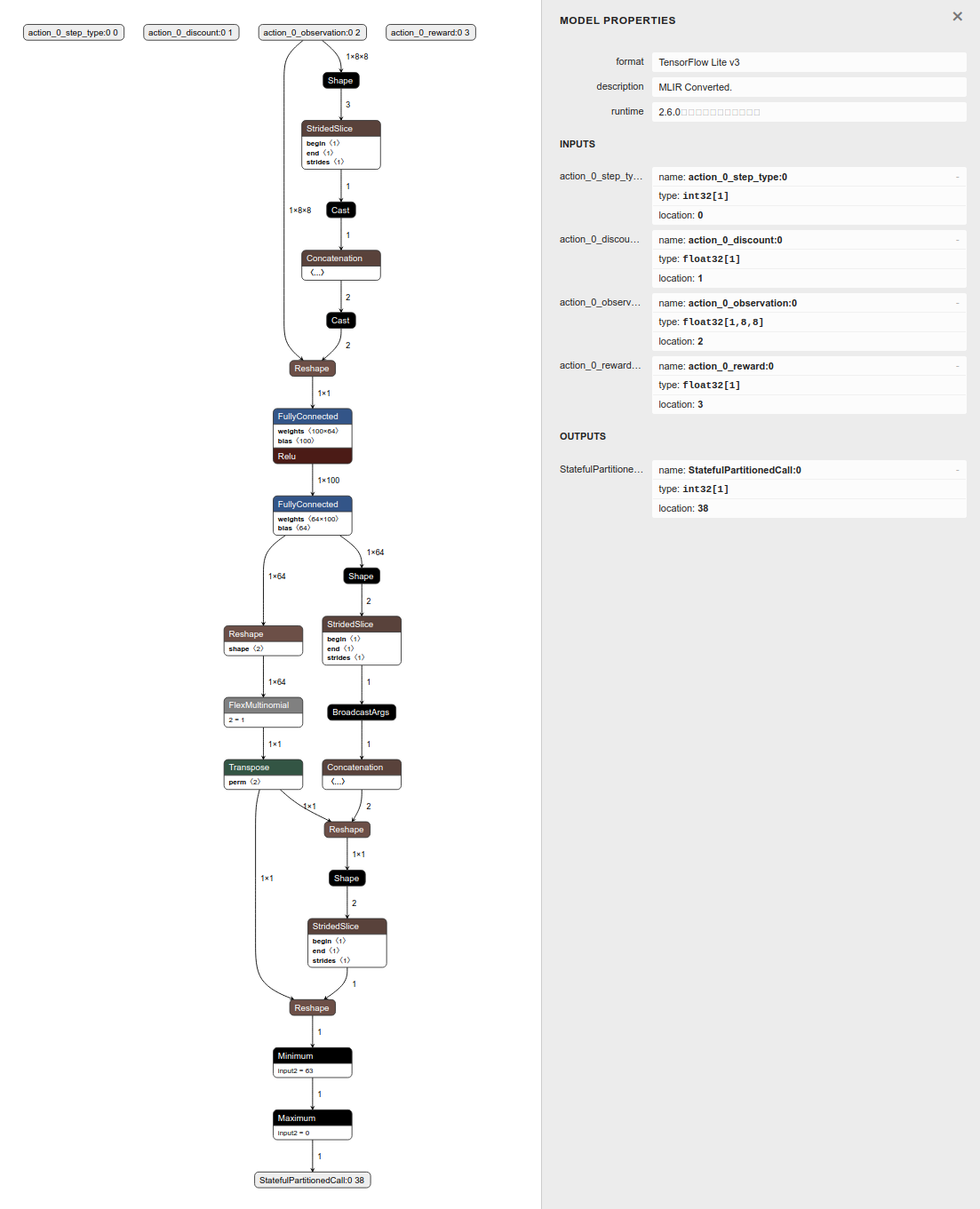Visualizing TFLite model converted from TF Agents using Netron

Also, the model directly outputs the strike position instead of the probability distribution, so we no longer need to do argmax manually.

``````@Override
protected void runInference() {
Map output = new HashMap<>();
// TF Agent directly returns the predicted action
int[][] prediction = new int;
output.put(0, prediction);
tflite.runForMultipleInputsOutputs(inputs, output);
agentStrikePosition = prediction;``````

So to summarize, in this post we showed you 2 paths of how to train a game agent, convert the trained model to TFLite and deploy it into an Android app. Hopefully this end-to-end tutorial helps you better understand how to leverage the TensorFlow ecosystem to build cool games.

And lastly, if this little game looks interesting to you, we challenge you to install the app on your phone and see if you can beat the agent we have trained 😃.

Next postPosted by Wei Wei, Developer Advocate Games are often used as test grounds for various reinforcement learning (RL) algorithms. While it is very exciting that machine learning researchers invent new RL algorithms to master challenging games, we are also curious to see that game developers are using RL to build gaming bots in TensorFlow for various purposes, such as quality testing, game balance tu…